# Curve Primitives

• A CurvePrimitive is a bounded continuous curve.
• All curves implement methods (e.g. `fractionToPoint` to refer to "fraction" position along the curve.
• `fraction=0` is the startof the primitive
• `fraction=1` is the end of the primitive
• increasing fractions always move forward along the primitive.
• curves implment their equations with the fraction representing the parameter in their most natural equations.
• All curves also support methods to deal with true distance along the curve. These include
• `curve.curveLengthBetweenFractions (startFraction, endFraction)`
• `curve.moveByDistanceFromFracton (startFracton, distance)`
• Fraction position along the curve is strictly proportional to true distance along the curve only for a limited number of curve types:
• LineSegment3d
• Arc3d
• TransitionSpiral
• Other curve types that have more complicated (non-proportional) fraction-to-distance relations are
• elliptic arcs
• bspline curves
• linestrings
• When movement "by distance" along a chain of curves (of varying types) is required, the `CurveChainWithDistanceIndex` will act like a single curve (starting and ending at fractions 0 and 1), with the fraction mapped to true distance along the chain.

## lineSegment

• A line segment is a portion of an infinite line.
• Json Fragment: `[{"lineSegment":[[0,0,0], [3,0,0]]}`
• typescript object:

``      const myLineSegment = LineSegment.create (Point3d.create (1,2,3), Point3d.create(6,4,2));``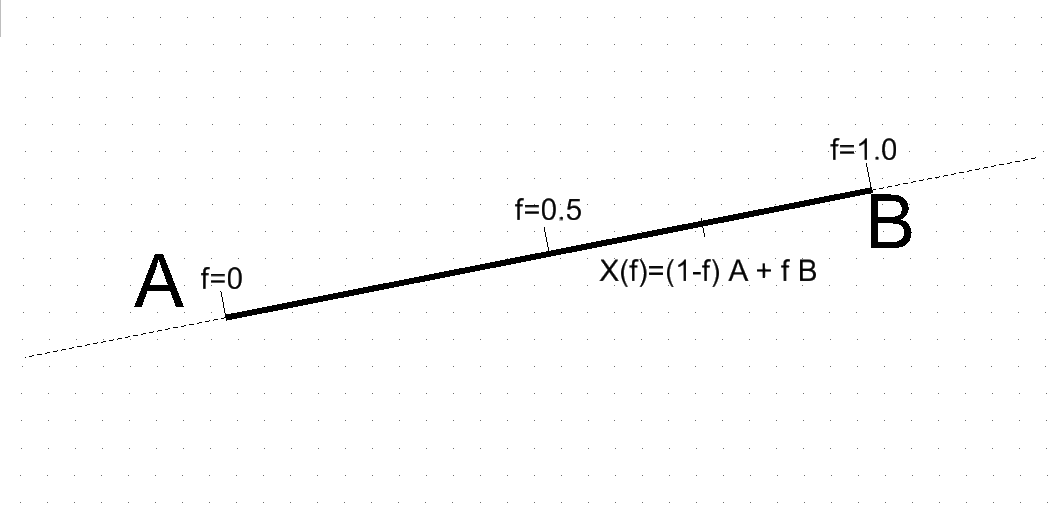• Fractional Parameterization:
``````A = start point
B = end point
f = fraction varying from 0 to 1
Point X(f) at fractional position f along the lineSegment is
X(f) = (1-f) * A + f * B``````

## lineString

• A LineString is an array of points that are to be connected by straight lines.
• Json Fragment: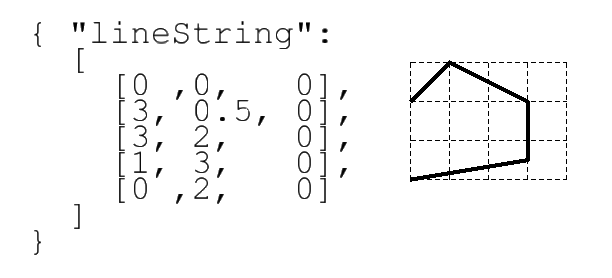• Typescript object:
``      const myLineString = LineString.create ([point0, point1, point2 ....]);``
• Fractional Parameterization

Having both individual line segments and the composite linestring complicates parameterization.

• As with all CurvePrimitives, the fractional parameterization for the complete linestring must have `fraction=0` at the start and `fraction=1` at the end.
• The fractional positions of each inerior vertex are then defined at equal intervals in the fraction space.
•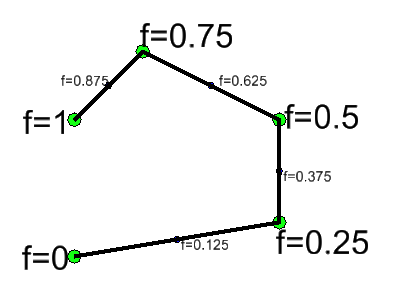• Hence in the example, with 4 segments the vertex fractions increment by one quarter.
• Within each segment, the fraction interval is mapped as if it were a line segment.
• Note that having uniform vertex-to-vertex fraction means that the distance-along-the-linestring is not proportional to fraction-along-entire-linestring. Fraction and distance changes are only proportional within individual segments.

## arcs (circular and elliptic)

An arc primitive is a portion of a circular or ellipticla arc. The equations for a complete elliptic arc require a center point and two vectors. The start and end of a partial arc are controlled by two angles.

The equational forms for circular and elliptic cases are identical. Telling whether a given arc is true circular requires examination of the vector coordinates.

The stroking equation that maps an angle to a coordinates to points around a (full) elliptic (or circular) arc is

``````    C = center point
U = vector from center point to 0-degere point
V = vector from center point to 90-degree point.
theta = angle
X(theta) = C + cos (theta * U + sin(theta) * V``````

### True Circles

• If the `U` and `V` vectors are (both) perpendicular and the same length, this is a true circle.
• In the both circles below, the `U` and `V` are identical length and perpendicular to each other.
• For the left circle, `U` and `V` happen to be in the global x and y directions.
• For the right circle, `U` and `V` are still identical length and perpendicular, but are both rotated away from global x and y. This still traces a circle, but the "0 degree" point is moved around the circle.
• When the circular arc conditions are true, the angle used in the equations is an the actual physical angle between the `U` vector and the vector from the center to `X(theta)`.### Ellipse

If the `U` and `V` vectors either (a) have different lengths or (b) are not perpendicular, the ellispe is non-circular.

If `U` and `V` are perpendicular, their lengths correspond to the common usage of "major" and "minor" axis lengths. But the perpendicular condition is not required -- non-perpendicular vectors occur due to transformation and construction history.

### Angular limits

To draw an arc that is not the complete circle or ellipse, simply limit the theta range to something other than 0 to 360 degrees.

``````    theta0 = angular start point
theta1 = angular and point
f = fraction varying from 0 to 1
theta(f) = (1-f) * theta0 + f * theta1
Point X(f) at fractional position f along the arc is

X(f) = C + cos (theta(f)) * U + sin(theta(f)) * V``````
• Angles theta0 and theta1 can be negative and can be outside of 360 degrees.
• Anlge theta1 can be less than theta0

Examples of arc sweep | start and end angles | CCW signed sweep angle | image | |----|----|---| | (0 to 360) | 360 |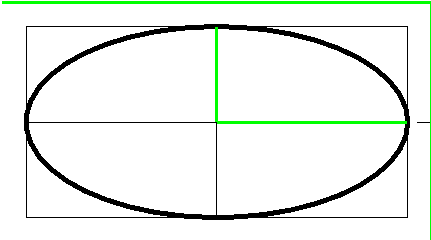| | (0 to 135) | 135 |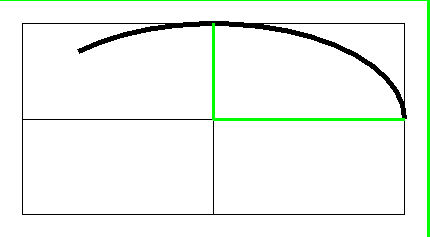| | (270 to 495) | 225 || | (90 to 270) | 180 |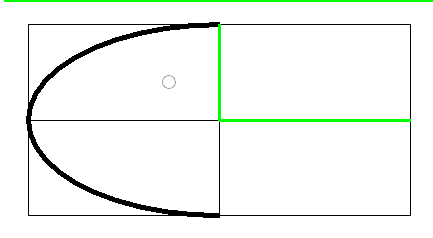| | (90 to 405) | 315 |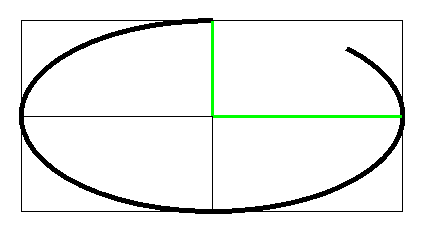|

Examples with json fragments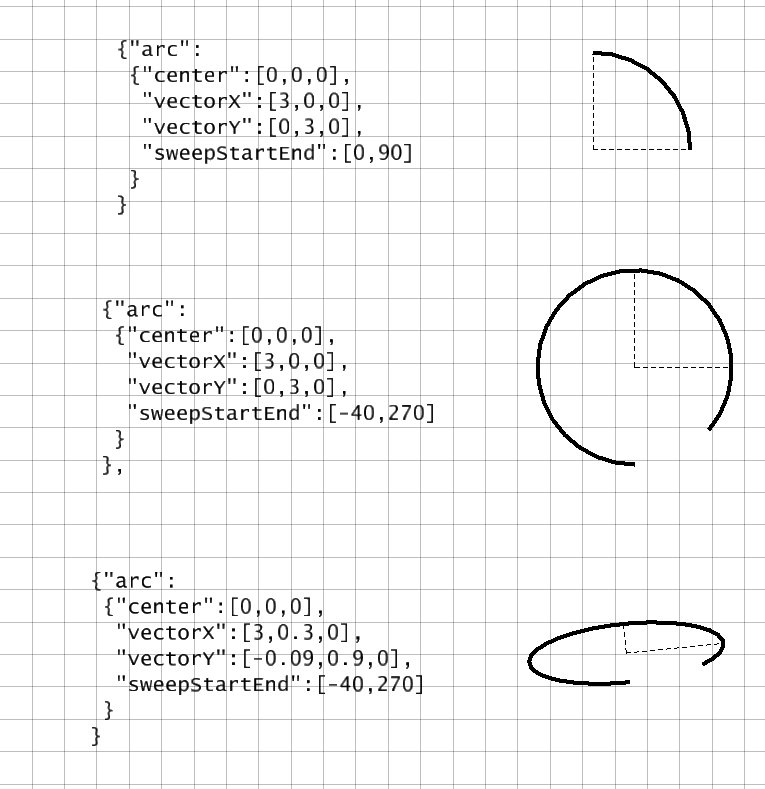# bspline curves

A BSplineCurve3d (or BSplineCurve3dH) is a curve that (loosely) follows a sequence of control points.

Internally, the curve is a is a sequence of polynomial curves that join together smoothly. Call each of those separate pieces a span.

The "control point" structure has remarkable properties for computation:

• The curve never leaves the overall xyz range of the control points.
• This bounding propery applies "from any veiwpoint", not just in the coordinate system where they are given.
• Even tighter, the curve is contained within the convex hull of the control points.
• No plane can intersect the curve more often than it intersects the control polygon.
• that is, the polygon may overestimate the number of intersections, (i.e. suggest false intersections), but it never underestimates.
• Inspection of the control polygon gives similar "never understimate" statements can be made about other propperties such as
• the number of inflections.
• the number of minima and maxima of the curve and its derivatives.
• The use of "weights" on the control points allows a bspline curve to exactly trace circular and elliptic arcs without use of trig functions.